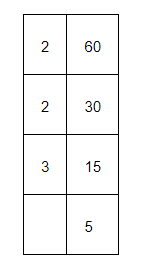# Write the following sets in roster form:

Question:

Write the following sets in roster form:

(i) $A=\{x: x$ is an integer and $-3 (ii)$\mathrm{B}=\{x: x$is a natural number less than 6$\}$. (iii)$C=\{x: x$is a two-digit natural number such that the sum of its digits is 8$\}$(iv)$D=\{x: x$is a prime number which is divisor of 60$\}$. (v)$E=$The set of all letters in the word TRIGONOMETRY. (vi)$F=$The set of all letters in the word BETTER. Solution: (i)$A=\{x: x$is an integer and$-3

The elements of this set are $-2,-1,0,1,2,3,4,5$, and 6 only.

Therefore, the given set can be written in roster form as

$A=\{-2,-1,0,1,2,3,4,5,6\}$

(ii) $B=\{x: x$ is a natural number less than 6$\}$

The elements of this set are $1,2,3,4$, and 5 only.

Therefore, the given set can be written in roster form as

$B=\{1,2,3,4,5\}$

(iii) $\mathrm{C}=\{x: x$ is a two-digit natural number such that the sum of its digits is 8$\}$

The elements of this set are $17,26,35,44,53,62,71$, and 80 only.

Therefore, this set can be written in roster form as

$C=\{17,26,35,44,53,62,71,80\}$

(iv) $D=\{x: x$ is a prime number which is a divisor of 60$\}$$\therefore 60=2 \times 2 \times 3 \times 5$

The elements of this set are 2, 3, and 5 only.

Therefore, this set can be written in roster form as D = {2, 3, 5}.

(v) E = The set of all letters in the word TRIGONOMETRY

There are 12 letters in the word TRIGONOMETRY, out of which letters T, R, and O are repeated.

Therefore, this set can be written in roster form as

E = {T, R, I, G, O, N, M, E, Y}

(vi) F = The set of all letters in the word BETTER

There are 6 letters in the word BETTER, out of which letters E and T are repeated.

Therefore, this set can be written in roster form as

F = {B, E, T, R}